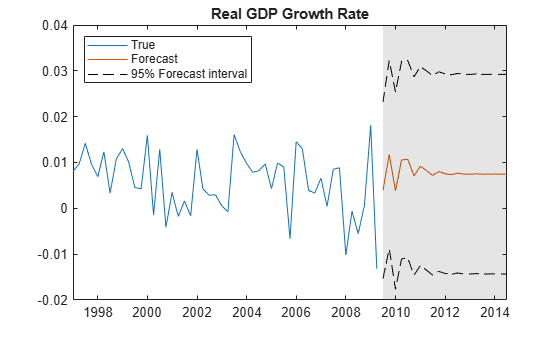# Forecast VAR Model

This example shows how to use `forecast` to forecast a VAR model.

`forecast` enables you to generate MMSE forecasts with error estimates. `forecast` requires:

• A fully-specified model (for example, `EstMdl` in what follows)

• The number of periods for the forecast (for example, `numperiods` in what follows)

• A presample time series (e.g., `Y(end-3:end,:)` in what follows)

`forecast` optionally takes:

• An exogenous data series

• Extra paths

Load the `Data_USEconModel` data set. This example uses two time series: the logarithm of real GDP, and the real 3-month T-bill rate, both differenced to be approximately stationary. Suppose that a VAR(4) model is appropriate to describe the time series.

```load Data_USEconModel DEF = log(DataTimeTable.CPIAUCSL); GDP = log(DataTimeTable.GDP); rGDP = diff(GDP - DEF); % Real GDP is GDP - deflation TB3 = 0.01*DataTimeTable.TB3MS; dDEF = 4*diff(DEF); % Scaling rTB3 = TB3(2:end) - dDEF; % Real interest is deflated Y = [rGDP,rTB3];```

Fit a VAR(4) model specification:

```Mdl = varm(2,4); Mdl.SeriesNames = {'Transformed real GDP','Transformed real 3-mo T-bill rate'}; EstMdl = estimate(Mdl,Y);```

Predict the evolution of the time series:

```numperiods = 21; FDates = dateshift(DataTimeTable.Time(end),'end','quarter',1:numperiods); [Forecast,ForecastMSE] = forecast(EstMdl,numperiods,Y(end-3:end,:));```

Extract the main diagonal elements from the matrices in each cell of `ForecastMSE`. Apply the square root of the result to obtain standard errors.

```extractMSE = @(x)diag(x)'; MSE = cellfun(extractMSE,ForecastMSE,'UniformOutput',false); SE = sqrt(cell2mat(MSE));```

Estimate approximate 95% forecast intervals for each response series.

```ForecastFI = zeros(numperiods,Mdl.NumSeries,2); ForecastFI(:,:,1) = Forecast - 2*SE; ForecastFI(:,:,2) = Forecast + 2*SE;```

Plot the forecasts and the data.

```figure h1 = plot(DataTimeTable.Time((end-49):end),Y((end-49):end,1)); hold on h2 = plot(FDates,Forecast(:,1)); h3 = plot(FDates,ForecastFI(:,1,1),'k--'); plot(FDates,ForecastFI(:,1,2),'k--'); title('Real GDP Growth Rate') h = gca; fill([FDates(1) h.XLim([2 2]) FDates(1)],h.YLim([1 1 2 2]),'k',... 'FaceAlpha',0.1,'EdgeColor','none'); legend([h1 h2 h3],'True','Forecast','95% Forecast interval',... 'Location','northwest') hold off``````figure h1 = plot(DataTimeTable.Time((end-49):end),Y((end-49):end,2)); hold on h2 = plot(FDates,Forecast(:,2)); h3 = plot(FDates,ForecastFI(:,2,1),'k--'); plot(FDates,ForecastFI(:,2,2),'k--'); title('3-month T-bill rate') h = gca; fill([FDates(1) h.XLim([2 2]) FDates(1)],h.YLim([1 1 2 2]),'k',... 'FaceAlpha',0.1,'EdgeColor','none'); legend([h1 h2 h3],'True','Forecast','95% Forecast interval',... 'Location','northwest') hold off```